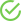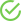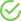Optimization

Starts from: \$19.99

Math optimization problems should really be called “projects,” for that is what they really are. They do not involve a math problem for which there is a single solution or method of arriving at a single solution. No, they involve finding multiple solutions and then determining which solution method is the best for a particular situation.

Features of the Optimization100% Original ProductComplete Money Back GuaranteesFREE AmendmentsPrivacy and Security

Math optimization problems should really be called “projects,” for that is what they really are. They do not involve a math problem for which there is a single solution or method of arriving at a single solution. No, they involve finding multiple solutions and then determining which solution method is the best for a particular situation.

### Understanding the Concept

The concept of math optimization is not difficult to understand. Basically, a math student is provided with a math problem. There may be several ways to solve that math problem, but which one is the best? Here is a simple example:

A student is asked to find the best way to determine 33% of a number. Now, those of us who remember basic math from late elementary school can probably think of at least three ways to solve this problem:

• We can multiply the given number, let’s say 60, by .33. With a trusty calculator, this is an easy solution. However, suppose we do not have a calculator or pencil and paper, and must figure this in our heads. We go to solution #2.
• We know what 10% of 60 is by moving the decimal point one place to the left. So 10% of 60 is 6. Now we can multiply that 6 by 3 in order to get 30%. That answer is 18. Now we just have to figure out what 3% of 60 is and add it to 18.  1% of 60 is found by moving the decimal point over one more place, or .6. Now, we multiply .6 times 3 and get 1.8. We add the 1.8 to 18 and get 19.8 for an answer.
• Still a third solution can be used if we don’t have to have the exact number. 33% of any number is roughly 1/3 of that number. So, 60 divided by 3 = 20. That’s pretty close to 19.8, the exact answer.

The second solution is obviously longer, but it would be the optimal solution if we did not have a calculator and needed the exact answer. The third solution is the optimal one if we did not have a calculator and did not need the exact answer. Each of these solutions is optimal for a specific situation, and that is really what math optimization is all about.

### When Optimization Becomes Complex

At the college level, students will obviously not be completing math optimization problems of finding per cents. No, when students are in college math courses, there are new “wrinkles,” such as the quantity to be optimized and the constraint quantity, and such. All of a sudden the concept becomes far more complex. Unfortunately, these types of problems are also included business, computer science, and economics classes too. So it is not just math and engineering students that get the pleasure of these projects.

### EssayRepublic.net Can Make “Light Work” of Your Optimization Projects

There is no reason to stress out over these problems/projects. We have mathematicians who love them, even though you do not. Send these problems over to us with our onsite order form, give us your deadline, and we will take care of the rest.

You’ll have a personal optimization expert who will come up with all of the possible solutions and make a recommendation for the best one based upon the situation you have been given. And, s/he will provide the justification that you will also need for selecting the solution you did. It really is that easy, and it is always confidential.

Once you have used our coursework assignment services, you will know that you can always depend on EssayRepublic.net for fast, professional, and original work.

On-time delivery

You can always rely on EssayRepublic.net! We, literally, never sleep and work day and night to deliver the best quality!

• Excuses? Never heard of them
• Fast and flawless? Yes we can!
Plagiarism-Free

You will never hear of plagiarism with EssayRepublic.net. The writers work exclusively from scratch!

Updated software to make sure the papers are plagiarism free

Ratings
Trustpilot

4.9

Sitejabber

4.75

Glassdoor

4.75

Manta

4.8